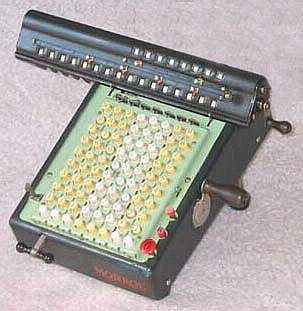previous <<==>> next

 MONROE LX-160 Have a look at a newer version``` T E C H N I C A L D A T A of the Mechanical Calculator MONROE LX-160 **************************************************************************** Portable with Hard Case ! Serial Number: 213070 ( green/black ) Dimensions: (ca.) Width = 11_1/2" / 29 cm Depth = 9_1/2" / 24 cm Height = 3_1/2" / 9 cm Weight: (ca.) 8 lbs / 3.7 kg = Machine 3_1/2 lbs / 1.6 kg = Hard Case Mechanics: 8*10 Full-Keyboard Split-Stepped Drum / Geteilte Staffelwalze  10s Carry Mechanism Counter Stepping Mechanism + Animation Functions: Add, Subtract, Multiply, Divide Registers: Input = 8 Decimals Counter = 8 Decimals (NO Carry; Neg.Figures RED) Arithmetic = 16 Decimals Manufacturer: MONROE Calculating Machine Co. New York / USA 1950 H O W T O U S E the MONROE LX-160 ***************************************** BEFORE STARTING A TASK: ----------------------- Turn MAIN CRANK in the top (idle) position. RELEASE the red REPEAT-Button (R) by pressing the red button () above. CLEAR the KEYBOARD by pressing the lower right red Button (0). CLEAR the COUNTER unit by turning the carriage crank clockwise. CLEAR the ARITHMETIC unit by turning the same crank counter-clockwise. Lift and shift the CARRIAGE to the far left ( = POSITION 1). ADDITION & SUBTRACTION: ----------------------- Example: 123 + 45 - 6 = 162 ADD: Enter the first number (123) in the far right columns. Make a positive (clockwise) turn with the main crank to transfer the number into arithmetic unit. The counting unit displays the figure 1. Enter the second number (45). Make a positive (clockwise) turn with the main crank to add the number. The arithmetic unit displays the intermediate sum (168) and the counting unit displays the figure 2. SUBTRACT: Enter the third number (6). Make a negative (counter-clockwise) turn with the main crank. The arithmetic unit displays the result (162) and the counting unit is decreased by 1. REMARK: NEGATIVE RESULTS are displayed in the arithmetic unit as the COMPLEMENT of the next higher 10, 100, 1000, ... Example: -12 = 99...9988 MULTIPLICATION: --------------- Example: 123 x 45 = 5535 Clear counter and arithmetic units. Press the right red REPEAT-Button (R). Enter the multiplicand (123) in the far right columns. The multiplicator (45) has two digits, so the arithmetic unit is moved to position 2 with a clockwise turn of the T-Handle in front. Repeat making positive (clockwise) turns with the main crank, until the first figure of the multiplicator (4) will appear in the 2nd position of the counter unit. Move the arithmetic unit with the T-Handle to position 1. Repeat making positive turns with the main crank, until the second figure of the multiplicator (5) appears in the 1st position of the counter unit. The multiplication is done: The multi- plicand (123) stays in keyboard, the multiplicator (45) in the counter unit and the result (5535) is in the arithmetic unit. DIVISION: --------- Example: 22 : 7 = 3.1428571 Remainder 3 Division requires 3 steps: (A) To Set the Dividend into Arithmetic Unit: ----------------------------------------- For the maximum number of decimals, pull out the arithmetic unit to the far right position. Enter the dividend (22) in the far right columns. Make a positive (clockwise) turn with the main crank to transfer into arithmetic unit. (B) To Set the Divisor in the Keyboard: ----------------------------------- Clear the counter unit by turning the carriage crank clockwise. Enter the divisor (7) below the dividend (22). Press the right red REPEAT-Button (R). (C) To Divide: ---------- Make negative (counter-clockwise) turns with the main crank until the arithmetic unit shows an "underflow". Make one positive (clockwise) turn with the main crank. Move the carriage with the T-handle to the next left position. Repeat this procedure until the required number of decimals are calculated... The result (3.1428571) is in the counter unit ( in RED ), and the remainder (3) is in the arithmetic unit. The divisor (7) stays in the input unit, therefore an additional decimal can be estimated ... ( 4, cause 4 x 7 = 28). REMARKS to the lower left "ITEM-COUNTER-CLIP": ============================================================================ Because the counter has no 10s-carry, there is no "build-in" counting of the items to add. Here the "Item-Counter-Clip" is useful: When this clip is switched right the (1) key is pressed, the arithmetic unit will count the items in this column(s)... In this case the "CLEAR KEYBOARD" Button will NOT clear this (1) key. Have a look at "Calculating Trickies" ... impressum: **************************************************************************** © C.HAMANN http://public.BHT-Berlin.de/hamann 01/02/07 ```